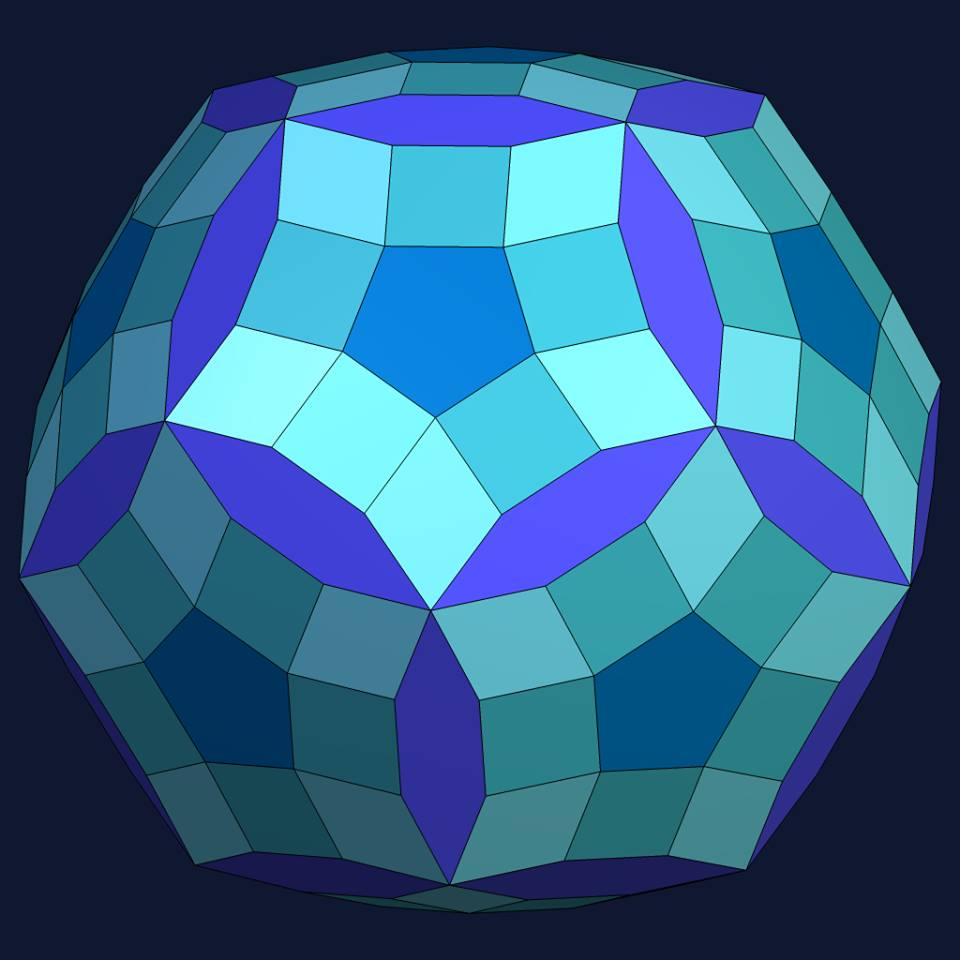Acronym ... Name s60-r60-p12-h30© Vertex figure [4,5,4,r], [4,R,H], [(r,h)3]

The rhombs {(r,R)2} have vertex angles r = arccos(1/3) = 70.528779° resp. R = arccos(-1/3) = 109.471221°. Esp. rr : RR = sqrt(2).
The hexagons {(h,H,H)2} have vertex angles h = arccos(sqrt(5)/3) = 41.810315° resp. H = arccos(-(1+sqrt(5))/sqrt(12)) = 159.094843°.

Incidence matrix

60   *  * |  2   2  0   0 |  1  2  1  0  [4,5,4,r]
* 120  * |  0   1  1   1 |  0  1  1  1  [4,R,H]
*   * 20 |  0   0  0   6 |  0  0  3  3  [(r,h)3]
----------+---------------+------------
2   0  0 | 60   *  *   * |  1  1  0  0
1   1  0 |  * 120  *   * |  0  1  1  0
0   2  0 |  *   * 60   * |  0  1  0  1
0   1  1 |  *   *  * 120 |  0  0  1  1
----------+---------------+------------
5   0  0 |  5   0  0   0 | 12  *  *  *  {5}
2   2  0 |  1   2  1   0 |  * 60  *  *  {4}
1   2  1 |  0   2  0   2 |  *  * 60  *  {(r,R)2}
0   4  2 |  0   0  2   4 |  *  *  * 30  {(h,H,H)2}